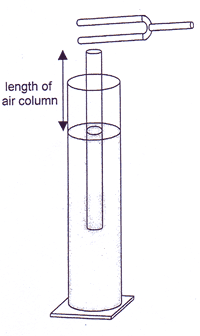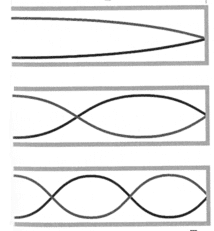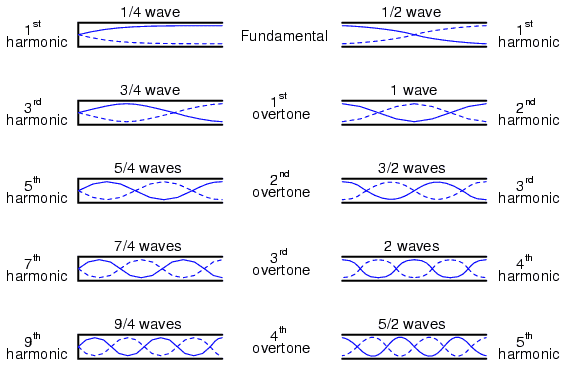# Sound waves in a closed pipe Problem

• pstir2
What is the speed of sound in air and the surrounding temperature?In summary, the student's experiment yielded results in which the frequency of the tuning fork and the length of the air column were both measured. The student also found that the temperature of the air was unknown.

## Homework Statement

Before I write the question you should know that my maths is all correct in my solution but I must have used the formulas incorrectly (or used the wrong formulas). I can't pinpoint where I've gone wrong or if I have left a formula out (I'm a teacher solving this question for an exam my students will be completing).

A student carries out an experiment to measure the speed of sound in air and its temperature. He uses the equipment shown at the right. The student measures the length of the air column when resonance is produced in the tube by the tuning fork.

Her results are listed below:
Frequency of Tuning Fork = 625Hz
Length of Air Column = 136mm = 0.136m
Temperature = ?a) At what length of pipe raised out of the water would the student obtain a second resonant point?

b) From the student’s results, what is the speed of sound in air and the surrounding temperature?
(Ignore end correction factors)

## Homework Equations

v = f.λ

λn=4L/(2n-1) (Closed Pipe)

Velocity of sound through air at different temperatures
vT=331√(T/273+1)

fn=nv/4L (Closed Pipe)

I have only included the closed pipe equations since this is a closed pipe question. If there are any other equations needed to solve this question please tell me.

## The Attempt at a Solution

a)second resonant is the middle pipe in this diagram (I think). There forethere the length of pipe out of the water is 3/4 of a wavelength.
λn=4L/(2n-1)
λ3=4x0.136/(2x3-1)
λ3=0.1088

L=3/4λ
L=3/4 x 0.1088
L=0.0816m

b)
v = f.λ
v = 625 x 0.1088
v = 68 m/s

vT=331√(T/273+1)
68 = 331√(T/273+1)
...
T = -261.47oC

pstir2 said:
He uses the equipment
pstir2 said:
Her results are listed below
The student appears to have some gender issues.
pstir2 said:
At what length of pipe raised out of the water would the student obtain a second resonant point?
Can't answer that unless we know that the given length is for the first resonant point.
pstir2 said:
λ3=4x0.136/(2x3-1)
isn't that the third resonant point? Where was that asked for?

pstir2 said:
v = 625 x 0.1088
I thought the 625Hz, 136mm was for the first resonant point, so λ=4L=544mm.

Dear PS,

Your second resonant calculation keeps L fixed and finds ##\lambda##. But the instruction asks for the second L with the same ##\lambda##. First L was at ##\lambda/4##, so second L will be at three times that.

BvU said:
Dear PS,

Your second resonant calculation keeps L fixed and finds ##\lambda##. But the instruction asks for the second L with the same ##\lambda##. First L was at ##\lambda/4##, so second L will be at three times that.
So you're saying my answer to part A is correct?

On the contrary.

pstir2 said:
So you're saying my answer to part A is correct?
I think BvU is agreeing with me that your calculation is wrong. Please explain why you calculate ##\lambda_3##, or indeed any wavelength other than ##\lambda_1##. There is only one wavelength involved, that corresponding to the tuning fork frequency and the speed of sound in air. But there are different values of L which will resonate with the fork.

BvU said:
Dear PS,

Your second resonant calculation keeps L fixed and finds ##\lambda##. But the instruction asks for the second L with the same ##\lambda##. First L was at ##\lambda/4##, so second L will be at three times that.
I thought that the formula that I used first in part A would take the available length of the pipe L = 0.136m and work out the length of it required to get a full wavelength. I then worked out 3/4 of a wavelength to find the length of pipe extended out of the water to get the third harmonic (I'm assuming nodes are resonant points, therefore the third harmonic of a closed pipe has 2 nodes, which like I said I think are resonant points).

If this is wrong please correct me. Like I said this is an area of physics that I am not an expert on. I know that there is/are error/s in my solution because when solving for the temperature it is -261 degrees Celsius which is way off from anything that could be considered reasonable. I'm thinking I have used the formulas incorrectly.So what does the "n" in that formula stand for?

I thought that the formula that I used first in part A would take the available length of the pipe L = 0.136m and work out the length of it required to get a full wavelength. I then worked out 3/4 of a wavelength to find the length of pipe extended out of the water to get the third harmonic
So full wavelenth is 0.544 m, right. That's settled then. Doesn't change anymore unless the speed of sound changes. If you then work out 3/4 of that to find the length of pipe extended out of the water to get the resonance corresponding to 3/4 ##\lambda##, that gives 3/4 ##\lambda ## = 0.408 m.

You confuse yourself bringing in the term third harmonic. For the tuning fork that would be 1875 Hz and you can bet that it's almost absent. For the pipe it so happens that it will also resonate (frequency-wise the pipe resonates at its second resonance: n = 2, L = 3/4 ##\lambda_{1825\;Hz}## in your formula) at this frequency, but it would be as good as unnoticeable (because of the dominance of the 1st resonance at 625 Hz).

Situation changes when the pipe is pulled out further: the second resonance (for the pipe) with the 625 Hz ground tone of the tuning fork has no competitors worth mentioning.

By the way: no tuning fork at hand do actually do the experiment ? Or a tone generator with a speaker ?

 Finally, I suspect the pictures distract you from the actual exercise too: imagine the second picture on the left, stretched in the horizontal direction by a factor of 3. That's what we are talking about: the second resonance for the pipe with this ##\lambda## that gave a first resonance at L = 0.136 m. Teaser: can you tell at what frequency such a long pipe would resonate for the first time

Last edited:
•pstir2
pstir2 said:
I thought that the formula that I used first in part A would take the available length of the pipe L = 0.136m and work out the length of it required to get a full wavelength. I then worked out 3/4 of a wavelength to find the length of pipe extended out of the water to get the third harmonic (I'm assuming nodes are resonant points, therefore the third harmonic of a closed pipe has 2 nodes, which like I said I think are resonant points).
But that is not what you did. The first thing you calculated was the wavelength of the third harmonic of the pipe at the initial length. I keep asking why you did that. Although it doesn't say, it seems that the given length corresponds to the fundamental.
Next, you took 3/4 of that to get the pipe length for which that wavelength would be the second harmonic. That would have been correct if you had calculated the wavelength correctly.

Last edited:
Well, at least you can't complain about a lack of interest and assistance from PF helpers :)

•pstir2
So you're saying the mathematical solution to the entire question would be:

a)
λn=4L/(2n-1)
λ1=4x0.136/(2x1-1)
λ1=0.544

L=3/4λ
L=3/4 x 0.544
L=0.408m

b)
fn=nv/4L
f1=(1 x v) /(4 x 0.136)
625=v/0.544
v=340m/s

vT=331√(T/273+1)
340 = 331√(T/273+1)
...
•